# 河南省产业结构演进和经济增长关系的实证分析——以1978~2017年时间序列数据为例 An Empirical Analysis of the Relationship between Industrial Structure Evolution and Economic Growth in Henan Province—Take the Time Sequence Data from 1978 to 2017

• 全文下载: PDF(605KB)    PP.48-59   DOI: 10.12677/SA.2019.81007
• 下载量: 296  浏览量: 1,725

Since the reform and opening up, economic development has been the focus of our work that is closely related to the national economy and people’s livelihood. After decades of development, our province has achieved remarkable economic development results. In recent years, the proposal of the new normal has revealed the current situation of China’s development at this stage and in the future. The party and government are also gradually focusing on changing the development mode and optimizing the industrial structure. This paper mainly studies the relationship between industrial structure and economic growth. First of all, it’s about the structure and economic growth of the industrial structure and the economy. Then the theory of mutual relation between them is discussed. According to the specific situation of Henan province, this paper discusses the achievements and problems of industrial structure and economic growth. Moreover, the relationship between the two has been empirically tested through the annual data from 1978 to 2017 since the reform and opening up. It shows that there is a long-term and stable equilibrium relationship between the change of industrial structure and economic growth. Industrial structure optimization can promote economic growth and each industry has different action mechanism to economic growth. Economic growth is counterproductive to industrial structure. In the conclusion, it analyzes the reason that the two can affect each other. Finally, aiming at the influence mechanism and deficiencies reflected by the empirical study, this paper proposes to improve the output value and mechanization level of the primary industry, promote the development of the secondary industry through new forms of industrialization and increase the proportion and quality of the tertiary industry.

1. 引言

2. 文献综述

2.1. 国外主要观点

2.2. 国内主要观点

3. 河南省经济增长现状

3.1. 经济增长总量变化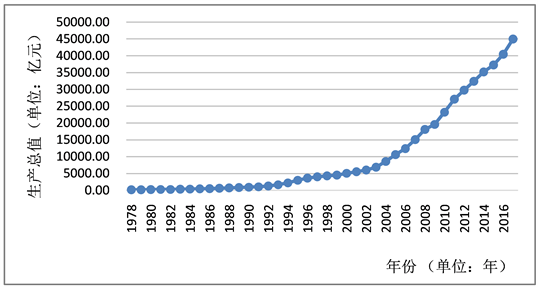注：根据河南省统计局网站和河南金融年鉴数据整理。

Figure 1. The change of the GDP of Henan province from 1978 to 2017

3.2. 经济增长速度变化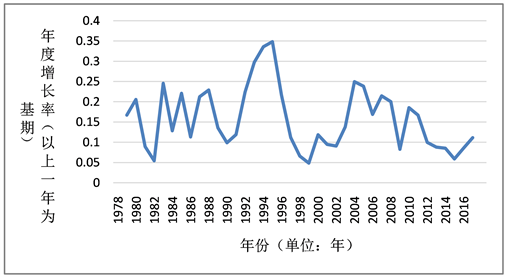注：根据河南省统计局网站和河南金融年鉴数据整理。

Figure 2. Changes of GDP growth rate of Henan province from 1978 to 2017

4. 河南省产业结构演变特点

4.1. 产业结构不断优化

4.1.1. 第一产业比重持续下降

4.1.2. 第二产业占比近年回落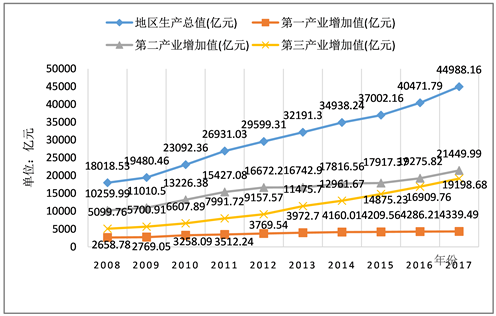注：根据河南省统计局网站数据整理。

Figure 3. Changes of GDP and added value of various industries in Henan province from 2008 to 2017注：根据河南统计局网站数据整理。

Figure 4. Changes of industrial structure in Henan province from 2008 to 2017

4.1.3. 第三产业发展势头强劲Table 1. Proportion of industrial structure in China and six provinces in central in 2017

4.1.4. 产业结构朝高级化演进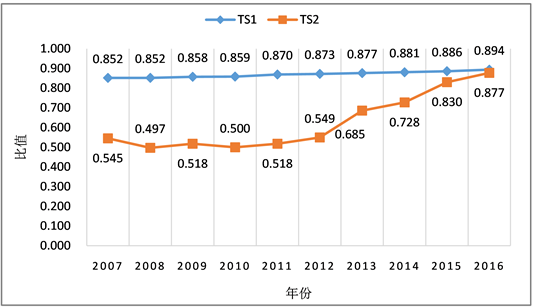注：根据河南省统计局网站数据整理。

Figure 5. Trend of TS1 and TS2

4.2. 产业结构调整过程中存在的问题

4.2.1. 产业发展整体低于全国平均水平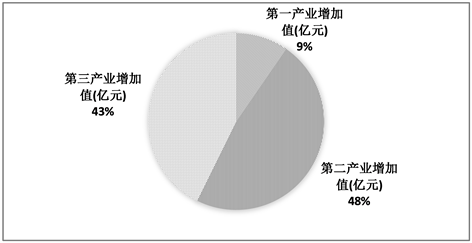注：根据河南省统计局网站数据整理。

Figure 6. Contribution ratio of three major industries to GDP of Henan province in 2017

4.2.2. 是第一产业生产效率较低

4.2.3. 是第二产业发展大而不强

4.2.4. 第三产业结构尚不合理

5. 实证分析

5.1. 数据选取和资料查找

5.2. 模型构建

$Y=A+{\alpha }_{1}{X}_{1}+{\alpha }_{2}{X}_{2}+{\alpha }_{3}{X}_{3}$

$LNY=C+{\beta }_{1}LN{X}_{1}+{\beta }_{2}LN{X}_{2}+{\beta }_{3}LN{X}_{3}$

5.3. ADF检验

5.4. 协整检验与回归Table 3. Cointegration test results of each variable

$\begin{array}{l}LNY=1.3459+0.1009LN{X}_{1}+0.5277LN{X}_{2}+0.3258LN{X}_{3}\\ t\text{ }\text{ }\text{ }\text{ }\text{ }\text{ }\text{ }=\text{ }\text{ }\text{ }\text{ }\text{ }\text{ }\text{ }\text{ }\text{ }\text{ }\left(14.7534\right)\text{ }\text{ }\text{ }\text{ }\text{ }\text{ }\text{ }\text{ }\text{ }\text{ }\text{ }\text{ }\text{ }\text{ }\text{ }\text{ }\text{ }\text{ }\text{ }\text{ }\text{ }\left(2.1729\right)\text{ }\text{ }\text{ }\text{ }\text{ }\text{ }\text{ }\text{ }\text{ }\text{ }\text{ }\text{ }\text{ }\text{ }\text{ }\text{ }\text{ }\text{ }\text{ }\text{ }\text{ }\text{ }\text{ }\text{ }\text{ }\left(18.3941\right)\text{ }\text{ }\text{ }\text{ }\text{ }\text{ }\text{ }\text{ }\text{ }\text{ }\text{ }\text{ }\text{ }\text{ }\text{ }\text{ }\text{ }\text{ }\text{ }\text{ }\text{ }\text{ }\text{\hspace{0.17em}}\text{\hspace{0.17em}}\text{ }\left(9.7747\right)\\ P=\text{ }\text{ }\text{ }\text{ }\text{ }\text{ }\text{ }\text{ }\text{ }\text{ }\text{ }\text{ }\left(0.0000\right)\text{ }\text{ }\text{ }\text{ }\text{ }\text{ }\text{ }\text{ }\text{ }\text{ }\text{ }\text{ }\text{ }\text{ }\text{ }\text{ }\text{ }\text{ }\text{ }\text{ }\text{ }\text{ }\text{ }\text{ }\left(0.0365\right)\text{ }\text{ }\text{ }\text{ }\text{ }\text{ }\text{ }\text{ }\text{ }\text{ }\text{ }\text{ }\text{ }\text{ }\text{ }\text{ }\text{ }\text{ }\text{ }\text{ }\text{ }\text{ }\text{ }\text{ }\text{ }\text{ }\text{ }\left(0.0000\right)\text{ }\text{ }\text{ }\text{ }\text{ }\text{ }\text{ }\text{ }\text{ }\text{ }\text{ }\text{ }\text{ }\text{ }\text{ }\text{ }\text{ }\text{ }\text{ }\text{ }\text{ }\text{ }\text{ }\text{ }\text{ }\text{ }\text{ }\text{\hspace{0.17em}}\text{ }\left(0.0000\right)\\ {R}^{2}=0.9998\text{ }\text{ }\text{ }\text{ }\text{ }\text{ }\text{ }\text{ }\text{ }\text{ }\text{ }\text{ }\text{ }\text{ }\text{ }\text{ }\text{ }\text{ }\text{ }\text{ }\text{ }F=54943.96\end{array}$

5.5. 格兰杰因果关系检验Table 4. Granger causality test results

5.6. 结论与对策

  Kuznets, S. (1949) National Income and Industrial Structure. Econometrica, 17, 205-241. https://doi.org/10.2307/1907310  Chenery, H.B. (1960) Patterns of Industrial Growth. American Economic Review, 50, 624-654.  O’Brien, A.M. (2002) Industrial Structure and Economic Growth. China Industrial Econo-my.  Fumitoshi, M., Tomoyasu, T., Noriyoshi, N., et al. (2018) Structural Reform of the Electricity Industry and Economic Growth. Journal of Economic Policy Reform, 1-25. https://doi.org/10.1080/17487870.2018.1469985  Laboure, M. and Taugourdeau, E. (2018) Does Government Expenditure Matter for Economic Growth? Global Policy, 9, 203-215. https://doi.org/10.1111/1758-5899.12540  朱嘉明. 速度•比例•结构——对我国经济发展速度和经济结构关系的探讨[J]. 中国社会科学院研究生院学报, 1979: 21-26.  刘伟, 张辉. 中国经济增长中的产业结构变迁和技术进步[J]. 经济研究, 2008(11): 4-15.  王蒙, 刘刚. 中国产业结构与经济增长研究: 一个经济增长分解框架[J]. 社会科学辑刊, 2017(4): 65-73.  王锋, 张芳, 刘娟. 产业结构对经济增长作用路径的实证检验[J]. 统计与决策, 2018(10): 135-138.  龚新蜀, 靳亚珍. 基于灰色关联理论的产业结构与经济协同发展的实证分析[J]. 统计与决策, 2018(2): 123-126.  刘建平, 王克林, 黄怀槐. 产业结构与经济增长关系的实证分析——以广东省为例[J]. 统计与决策, 2006(2): 70-72.